# Mohr's Circle for 2-D Stress Analysis

If you want to know the principal stresses and maximum shear stresses, you can simply make it through 2-D or 3-D Mohr's cirlcles!

You can know about the theory of  Mohr's circles from any text books of Mechanics of Materials. The following two are good references, for examples.

1.  Ferdinand P. Beer and E. Russell Johnson, Jr, "Mechanics of Materials", Second Edition, McGraw-Hill, Inc, 1992.
2 . James M. Gere and Stephen P. Timoshenko, "Mechanics of Materials", Third Edition, PWS-KENT Publishing Company, Boston, 1990.

The 2-D stresses, so called plane stress problem, are usually given by the three stress components  sx , sy , and txy ,  which consist in a  two-by-two symmetric matrix (stress tensor):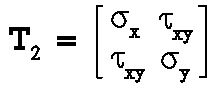(1)
What people usually are interested in more are the two prinicipal stresses s1 and s2 , which are the two eigenvalues of the two-by-two symmetric matrix of Eqn (1), and  the maximum shear stress tmax , which can be calculated from s1 and s2 .  Now, see  the Fig. 1 below, which represents that a state of plane stress exists at point O and that it is defined by the stress components  sx , sy , and txy  associated with the left element in the Fig. 1. We  propose to determine the stress components sxq , syq , and txyq    associated with the right element after it has been rotated through an angle q about the z axis.Fig. 1  Plane stresses in different orientations

Then, we have the following relationship:

sx= sx cos 2 q + sy sin2 q + 2 txy sin q cos q
(2)
and
txy= -(s- sy ) cos2 q +  txy (cos2 q - sin2 q)
(3)
Equivalently, the above two equations can be rewritten as follows:
sx= (sx sy)/2 + (sx sy)/2 cos 2q + txy sin 2q
(4)
and
txy= -(sx sy)/2 sin 2q + txy cos 2q
(5)
The expression for the normal stress syq may  be obtained by replacing the  q in the relation for sxq in Eqn. 3 by q + 90o  ,  it turns out to be
sy= (sx sy)/2 - (sx sy)/2 cos 2q - txy sin 2q
(6)
From the  relations for sxq and syq , one obtains the circle equation:
(sxq - save)2   + t2xyq  = R2m
(7)

where
save = (sx sy)/2  = (sxq syq)/2 ;    Rm =  [(sx sy)2 / 4 + t2xy]1/2
(8)
This circle is with radius  R2m and centered at  C = (save  ,  0)  if  let s = sxq  and  t = -txyas shown in  Fig. 2 below - that is right the Mohr's Circle  for plane stress problem  or 2-D stress problem!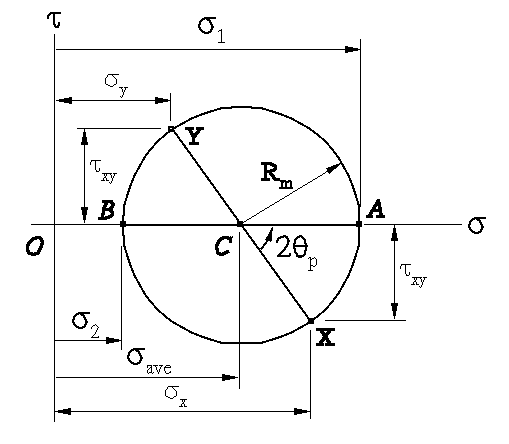Fig. 2  Mohr's circle for plane (2-D) stress

In fact, Eqns. 4 and 5 are the parametric equations for the Mohr's circle!  In  Fig. 2, one reads  that  the point

X = (sx , -txy )
(9)
which corresponds to the point at which q = 0 and the point
A = (s1 , 0 )
(10)
which corresponds to the point at which q = qp that gives the principal stress s1 ! Note that
tan 2qp = 2txy /(sx sy)
(11)
and the point
Y = (sy , txy )
(12)
which corresponds to the point at which q = 90o and the point
B = (s2 , 0 )
(13)
which corresponds to the point at which q = qp + 90o that gives the principal stress s2 !  To this end, one can pick the maxium normal stressess as
smax = max(s1 , s2),  smin = min(s1 , s2)
(14)
Besides, finally one can also read the maxium shear stress as
tmax = Rm =  [(sx sy)2 / 4 + t2xy]1/2
(15)
which corresponds to the apex of the Mohr's circle at which q = qp + 45o !
(The end.)

# Mohr's Circles for 3-D Stress Analysis

The 3-D stresses, so called spatial stress problem,  are usually given by the six stress components sx , sy , sz  , txy  , tyz  , and tzx  , (see Fig. 3) which consist in a three-by-three symmetric matrix (stress tensor):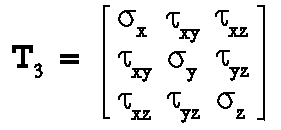(16)
What people usually are interested in more are the three prinicipal stresses s1 , s2 , and s3 , which are eigenvalues of the  three-by-three symmetric matrix of Eqn (16) ,  and the three maximum shear stresses tmax1 , tmax2 , and tmax3 which can be calculated from s1 , s2 , and s3 .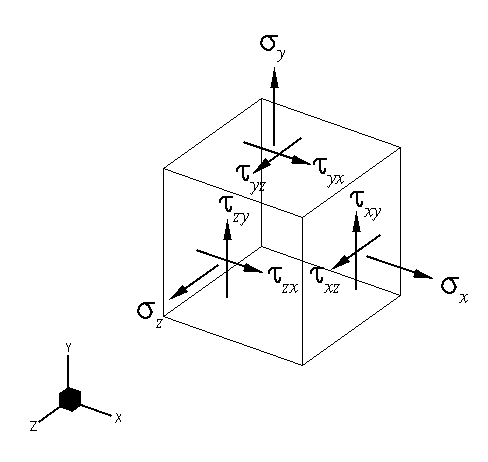Fig. 3  3-D stress state represented by axes parallel to X-Y-Z

Imagine that there is a plane cut through the cube in Fig. 3 , and the unit normal vector  n of  the cut plane has the direction cosines vx , vy , and vz , that is

n = (vx , vy , vz)
(17)
then the normal stress on this plane can be represented by
sn  = sxv2x + syv2y + szv2z + 2 txyvxvy + 2 tyzvyvz + 2 txzvxvz
(18)
There exist three sets of direction cosines, n1, n2, and n3 - the three principal axes, which make sn achieve extreme values s1 , s2 , and s3 - the three principal stresses, and on the corresponding cut planes, the shear stresses vanish!  The problem of finding the principal stresses and their associated axes is equivalent to finding the eigenvalues and eigenvectors of the following problem:
(sI3 - T3)n = 0
(19)
The three eigenvalues of Eqn (19) are the roots of  the following characteristic polynomial equation:
det(sI3 - T3) = s3 - As2 + Bs - C = 0
(20)
where
A = sx + sy + sz
(21)
B = sxsy + sysz + sxs - t2xy - t2yz - t2xz
(22)
C = sxsysz + 2 txytyztxz - sxt2yz- syt2xz- szt2xy
(23)
In fact,  the coefficients A, B, and C in Eqn (20) are invariants as long as the stress state is prescribed(see e.g. Ref. 2) . Therefore, if the three roots of Eqn (20) are s1, s2 , and s3 , one has the following equations:
s1 + s2 + s3 = A
(24)
s1s2 + s2s3 + s1s3 = B
(25)
s1s2s= C
(26)
Numerically, one can always find one of the three roots of Eqn (20) , e.g.  s1 , using line search algorithm, e.g. bisection  algorithm. Then combining Eqns (24)and (25),  one obtains a simple quadratic equations and therefore obtains two other roots of Eqn (20),  e.g. s2 and s3 .  To this end, one can re-order the three roots and obtains the three principal stresses, e.g.
s1 = max(s1 , s2 , s3)
(27)
s3 = min(s1 , s2 , s3)
(28)
s2 = (A - s1 - s2 )
(29)

Now, substituting s1 , s2 , or s into Eqn (19), one can obtains the corresponding principal axes n1, n2, or n3 , respectively.

Similar to Fig. 3,  one can imagine a cube with their faces normal to n1, n2, or n3 . For example, one can do so in Fig. 3 by replacing the axes X,Y, and Z with n1, n2, and n3 , respectively,  replacing  the normal stresses sx , sy , and sz  with the principal stresses s1 , s2 , and s3 , respectively, and removing the shear stresses txy , tyz , and tzx .

Now,  pay attention the new cube with axes n1, n2, and n3 . Let the cube be rotated about the axis n3 , then the corresponding transformation of stress may be analyzed by means of Mohr's circle as if it were a transformation of plane stress. Indeed, the shear stresses excerted on the faces normal to the n3 axis remain equal to zero, and the normal stress sis perpendicular to the plane spanned by n1 and n2 in which the transformation takes place and thus, does not affect this transformation.  One may therefore use the circle of diameter AB to determine the normal and shear stresses exerted on the faces of the cube as it is rotated about the  n3 axis (see Fig. 4). Similarly, the circles of diameter BC and CA may be used to determine the stresses on the cube as it is rotated about the n1 and n axes, respectively.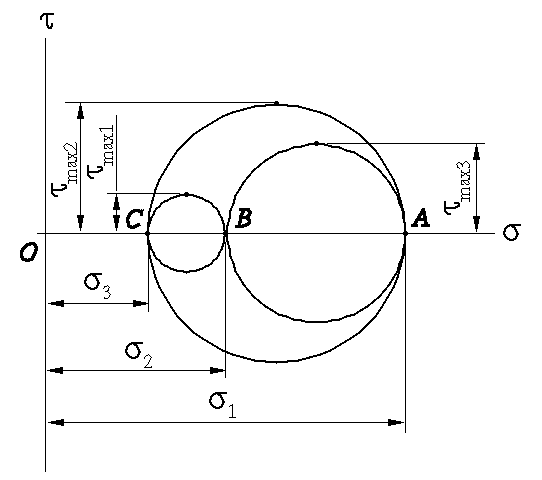Fig. 4  Mohr's circles for space (3-D) stress

What if the rotations are about the axes rather than principal axes? It can be shown that any other transformation of axes would lead to stresses represented in Fig. 4 by a point located within the area which is bounded by the bigest circle with the other two circles removed!

Therefore,  one can obtain the maxium/minimum normal and shear stresses from Mohr's circles for 3-D stress as shown in  Fig. 4!

Note the notations above (which may be different from other references), one obtains that

smax  =  s1
(30)
smin  =  s3
(31)
tmax  = (s- s3)/2 = tmax2
(32)
Note that in Fig. 4,  tmax1 , tmax2 , and  tmax3  are the maximum shear stresses obtained while the rotation is about   n1, n2, and n , respectively.
(The end.)

# Mohr's Circles for Strain and for Moments and Products of Inertia

Mohr's circle(s) can be used for strain analysis and for moments and products of inertia  and other quantities as long as they can be represented by two-by-two or three-by-three symmetric matrices (tensors).
(The end.)